QuestionAnswers

# Draw a line segment of length 7.6 cm and construct its perpendicular bisector.Verified
113.1K+ Views
Hint: In this question, we will first draw a line segment of given length and then construct arcs from its two end points and join the point of intersection of arcs to draw its perpendicular bisector.

Firstly, let us draw a line segment AB of length 7.6 cm with ruler scale.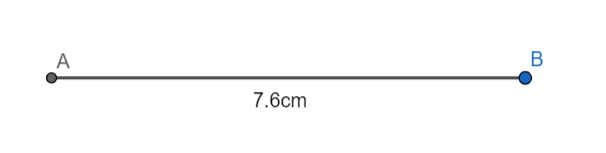Now, let us take a compass and open it to a radius which is more than half of the length of the line segment and less than full length of line segment.
Let us now place the compass at point A and draw an arc above the line segment.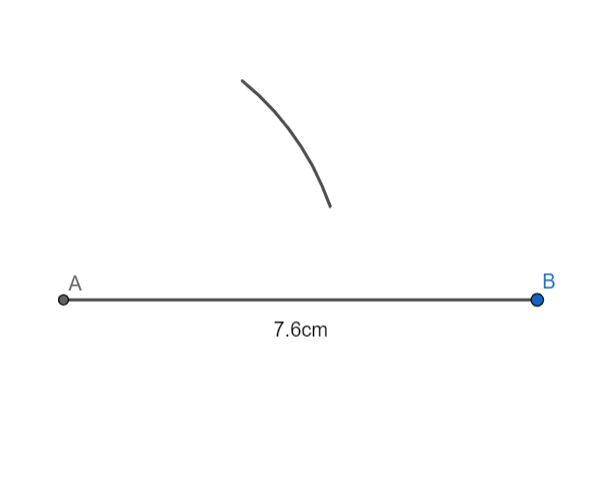Now, without changing the radius of the compass, we will draw an arc from point A again, below the line segment.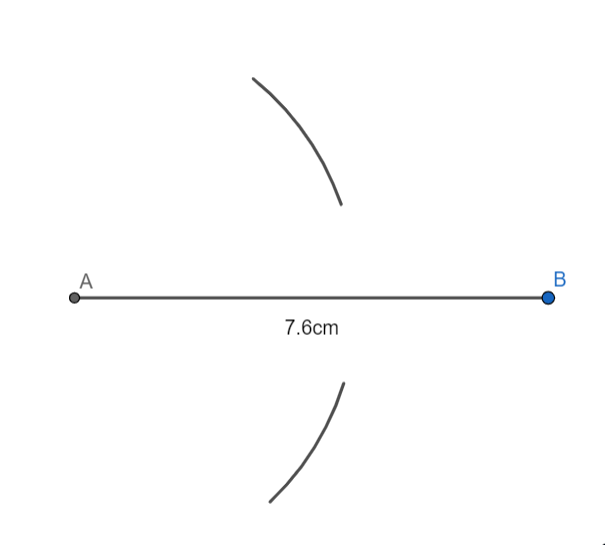We will now repeat the same with point B.
Without changing the radius of the compass, let us now place it at point B and draw an arc above the line segment.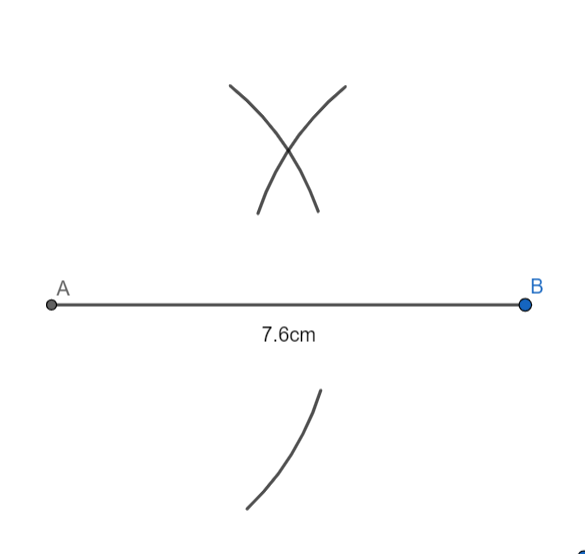Now, with the same radius of the compass, we will draw an arc from point B again, below the line segment.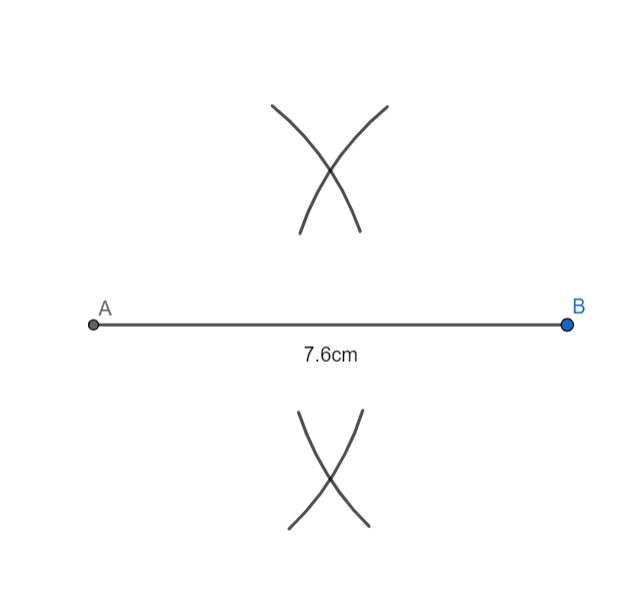We will mark the points of intersection of arcs above the line segment and below the line segment as R and S.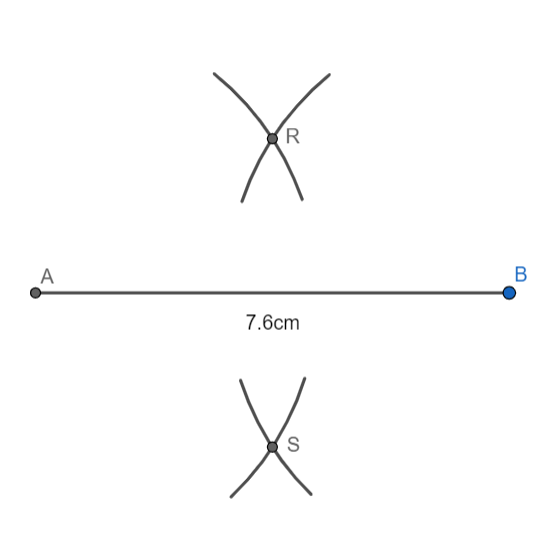Now, we will draw a straight line passing through points R and S and mark it UT.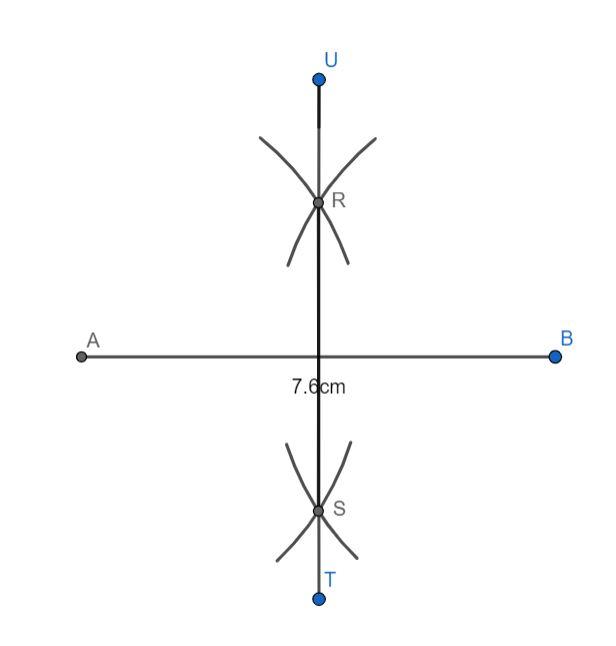Hence, UT is a perpendicular bisector of line segment AB of length 7.6 cm.

Note: While drawing arcs, check that the tip of the compass does not move from points A or B. Also, once you have taken the radius of the compass to draw an arc, do not change it in the whole construction procedure.